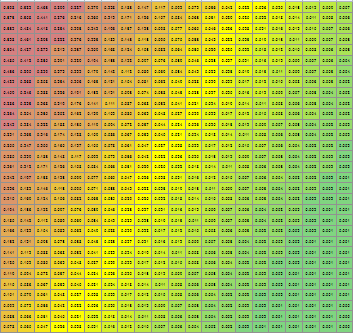## PROC LOGISTIC plots

hello all,

I am running this PROC LOGISTIC:

proc logistic data=expi2 plots=effect ( clband=yes SHOWOBS=NO ) ;
model criterion (event= '1') = predictor1 predictor2;

the PLOTS=EFFECT option gives me the plot for predictor1, but not for predictor2.

Is there a way to obtain a plot with both predictors?

I have seen @Rick_SAS  syntax for plotting different groups in the same graph, but I have not found a solution for what I need.

The datafile is attached.

Thanks in advance for any suggestions you may have.

Eman

1 ACCEPTED SOLUTION

Accepted Solutions

## Re: PROC LOGISTIC plots

Producing a 3D plot for two continuous variables is very easy - in fact, it is the default of the EFFECTPLOT statement in that situation. The following produces a contour plot that should be pretty straightforward for any audience to understand.

``````proc logistic data=expi2;
model criterion (event= '1') = predictor1 predictor2 ;
effectplot;
run;``````
12 REPLIES 12

## Re: PROC LOGISTIC plots

Of course, I can use the store option and PLM, but that still gives me two separate plots, one for each predictor.

proc logistic data=expi2 plots=effect ( clband=yes SHOWOBS=NO ) ;
model criterion (event= '1') = predictor1 predictor2 ;

store pip;

proc plm restore=pip;
effectplot fit (x=predictor1 );
effectplot fit (x=predictor2 );

## Re: PROC LOGISTIC plots

``proc logistic data=expi2 plots=effect ( clband=yes SHOWOBS=NO x=(predictor1 predictor2)) ;``

By the way, you did not attach the data and in fact, you should NOT attach data. Data should be provided as working SAS data step code (examples and instructions) and not via file attachments or screen captures.

--
Paige Miller

## Re: PROC LOGISTIC plots

hello.

What you suggest produces two separate graphs, one for predictor1 and one for predictor2.

The same results than with proc plm -- but your solution is of course more efficient.

What I would like is to have slopes for both predictors in one graph.

Eman

## Re: PROC LOGISTIC plots

I am not aware of any built-in way to have both EFFECT plots on one graph. It doesn't even make sense to do this unless PREDICTOR1 and PREDICTOR2 are on the exact same scale, otherwise you would need two different x-axes.

Of course, you can write your own program to put them on the same graph.

--
Paige Miller

## Re: PROC LOGISTIC plots

yes, they are on the exact same scale, that is why I would like to put them on the same graph.

## Re: PROC LOGISTIC plots

When the admittedly limited options of PLOTS options in an analysis procedure do not create the graphs you want then the approach means export the needed data from the procedure and write your own plot(s) with Proc Sgplot or Sgpanel or even the Graph Template Language and Proc Sgrender.

## Re: PROC LOGISTIC plots

You need to realize that with 2 (or more) x-variables, the only way to do this is to hold X1 constant and then get prediction curve for the X2. And then repeat the process holding the other X2 constant and predict X1. Which may or may not be what you are hoping to get from such a plot. Maybe what you really want is a 3-D plot of probabilities with axes X1 and X2 and predicted probabilities, but I'd have to think of how you could create such a plot. I have done a similar thing using PROC REPORT, showing probabilities in a matrix of X1 and X2, but this can't be called a "plot".

--
Paige Miller

## Re: PROC LOGISTIC plots

I will look into that, although a 3-D plot might be too complicated to read for my audience.

Yes, what you suggest is what I have in mind. I can reorganise my data and obtain the same through other means.

I was just curious if PROC LOGISTIC could handle it itself, but I guess this is not the case. Admittedly, it is not something that is likely to be useful very often, so I am not surprised that it does not exist as a built-in capability.

Thank you all for engaging in the discussion.

## Re: PROC LOGISTIC plots

Producing a 3D plot for two continuous variables is very easy - in fact, it is the default of the EFFECTPLOT statement in that situation. The following produces a contour plot that should be pretty straightforward for any audience to understand.

``````proc logistic data=expi2;
model criterion (event= '1') = predictor1 predictor2 ;
effectplot;
run;``````

## Re: PROC LOGISTIC plots

That is true, it is not that difficult. I will go with this!

## Re: PROC LOGISTIC plots

Sample of a PROC REPORT output for probabilities of two variables, colored (red=high prob, green=low prob) to make it more like a "plot". I have removed the column and row headers for confidentiality. Also, in the real PROC REPORT output, the numbers in the cells are large enough to be readable.--
Paige Miller

## Re: PROC LOGISTIC plots

This looks awesome indeed.

I have never used PROC REPORT, but I will look into it.

Thank you!

Discussion stats
• 12 replies
• 600 views
• 1 like
• 4 in conversation